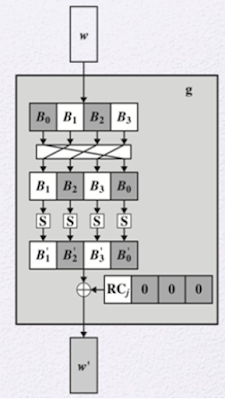Wednesday, October 13, 2021

Key Exapansion in AES | Add Round Key Function in AES | How key expnasion function works in AES Add Round Key?

Key Expansion in AES

The AES algorithm which takes 128 bits (16 bytes) key as input, four-word key as input and produce a linear array of 44 words. (In AES 4 Bytes = 1 Word). Figure describe the steps of key expansion.Figure :  1. Four word round 2. AES Encryption Process

A four-word round key is acceptable for the initial stage. The initial stage is Add Round Key and each of the 10 rounds of cipher. So, total 11 rounds = 44 words. The key is added into the first four words of the expanded key.Figure : Function g

The function g defines the complex and that function consist of some sub function as follow:

Step-1: This function performs the one-byte circular left shift.

Step-2: Using S-box each sub word performs a byte substitution.

Step-3: Finally result of Rot word and step 2 is XORed with the round constant called as Rconst[j].Figure : Key Expansion process in AES